Journal of Integer Sequences, Vol. 13 (2010), Article 10.5.4

## Catalan Numbers Modulo 2k

### Shu-Chung Liu Department of Applied Mathematics National Hsinchu University of Education Hsinchu, Taiwan and Jean C.-C. Yeh Department of Mathematics Texas A & M University College Station, TX 77843-3368 USA

Abstract:

In this paper, we develop a systematic tool to calculate the congruences of some combinatorial numbers involving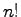. Using this tool, we re-prove Kummer's and Lucas' theorems in a unique concept, and classify the congruences of the Catalan numbers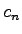(mod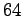). To achieve the second goal,(mod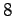) and(mod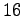) are also classified. Through the approach of these three congruence problems, we develop several general properties. For instance, a general formula with powers of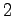and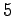can evaluate(mod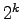) for any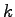. An equivalence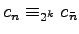is derived, where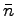is the number obtained by partially truncating some runs of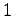and runs of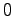in the binary string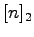. By this equivalence relation, we show that not every number in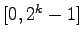turns out to be a residue of(mod) for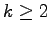.

Full version:  pdf,    dvi,    ps,    latex

Received January 14 2010; revised version received April 30 2010. Published in Journal of Integer Sequences, May 3 2010.